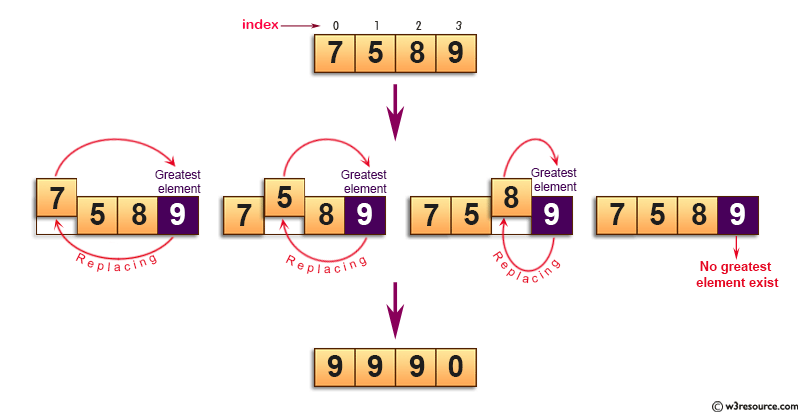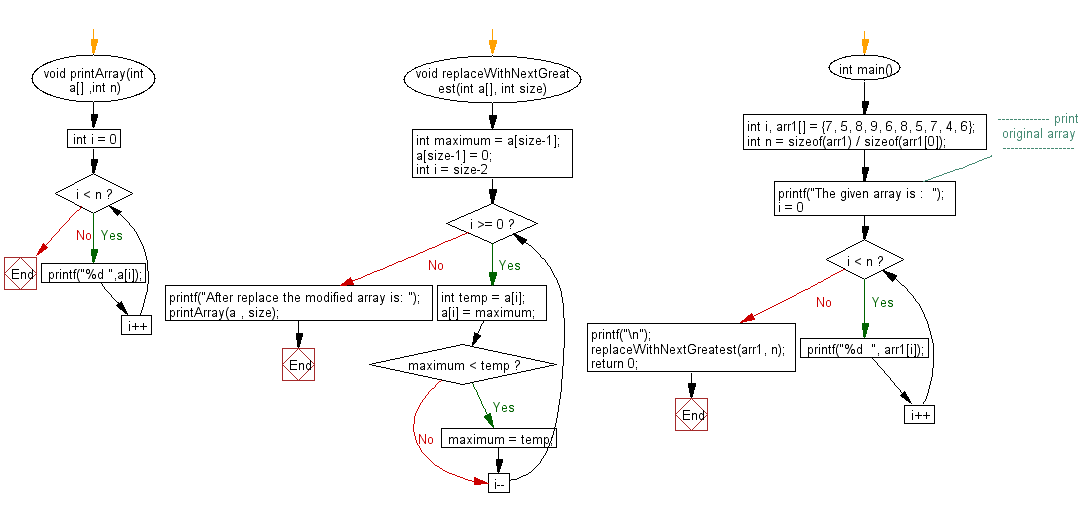﻿ C exercises: Replace every element with the greatest element on its right side - w3resource# C Exercises: Replace every element with the greatest element on its right side

## C Array: Exercise-63 with Solution

Write a program in C to replace every element with the greatest element on its right side.

Pictorial Presentation:Sample Solution:

C Code:

``````#include <stdio.h>

void printArray(int a[] ,int n)
{
for(int i = 0;i < n;i++)
printf("%d ",a[i]);
}

void replaceWithNextGreatest(int a[], int size)
{
int maximum =  a[size-1];
a[size-1] = 0;
for(int i = size-2; i >= 0; i--)
{
int temp = a[i];
a[i] = maximum;
if(maximum < temp)
maximum = temp;
}
printf("After replace the modified array is: ");
printArray(a , size);
}

int main()
{
int i, arr1[] = {7, 5, 8, 9, 6, 8, 5, 7, 4, 6};
int n = sizeof(arr1) / sizeof(arr1);
//------------- print original array ------------------
printf("The given array is :  ");
for(i = 0; i < n; i++)
{
printf("%d  ", arr1[i]);
}
printf("\n");
//------------------------------------------------------
replaceWithNextGreatest(arr1, n);
return 0;
}
```
```

Sample Output:

```The given array is :  7  5  8  9  6  8  5  7  4  6
After replace the modified array is: 9 9 9 8 8 7 7 6 6 0
```

Flowchart:C Programming Code Editor:

Improve this sample solution and post your code through Disqus.

What is the difficulty level of this exercise?

﻿